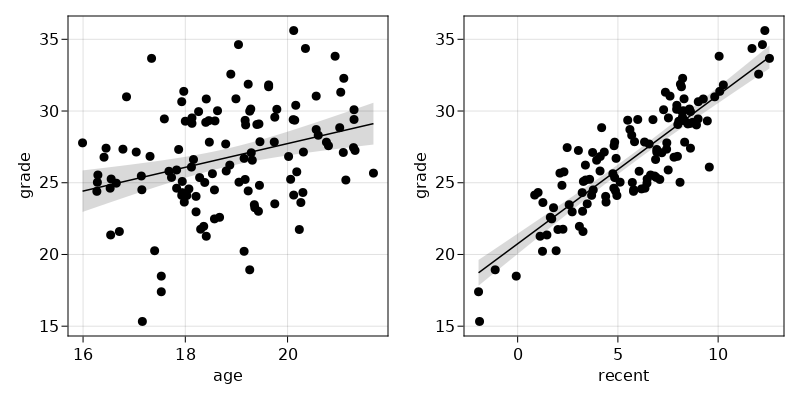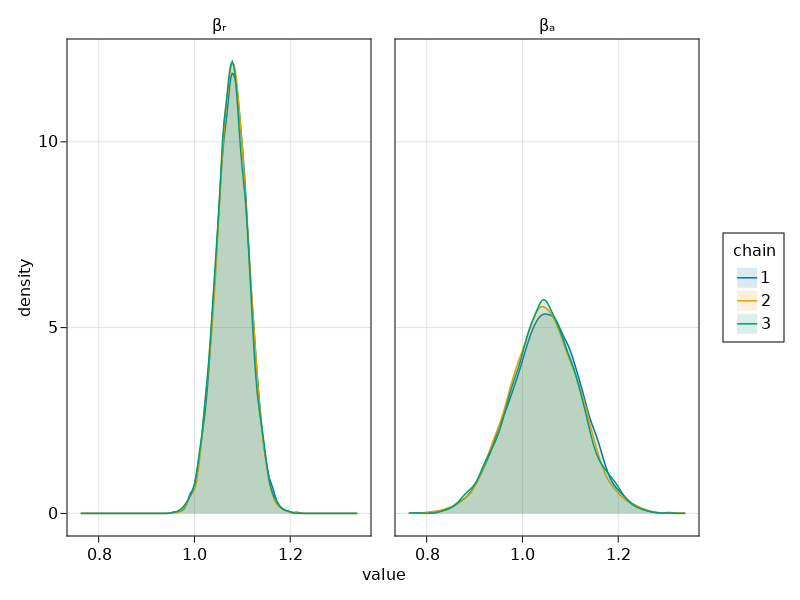Typically, when making predictions via a linear model, we fit the model on our data and make predictions from the fitted model. However, this doesn't take much foreknowledge into account. For example, when predicting a person's length given only the weight and gender, we already have an intuition about the effect size and direction. Bayesian analysis should be able to incorporate this prior information.

In this blog post, I aim to figure out whether foreknowledge can, in theory, increase model accuracy. To do this, I generate data and fit a linear model and a Bayesian binary regression. Next, I compare the accuracy of the model parameters from the linear and Bayesian model.

begin
using AlgebraOfGraphics
using CairoMakie
using CategoricalArrays
using DataFrames
using GLM
using MLDataUtils: rescale!
using Random: seed!
using Statistics
using StatsFuns
using Turing
end

We define the model as $g_i = a_e * a_i + r_e * r_i + \epsilon_i = 1.1 * a_i + 1.05 * r_i + \epsilon_i$ where $a_e$ is the coefficient for the age, $r_e$ is a coefficient for the nationality and $\epsilon_i$ is some random noise for individual $i$.

We generate data for $n$ individuals via:

function generate_data(i::Int)
seed!(i)

n = 120
I = 1:n
P = [i % 2 == 0 for i in I]
r_2(x) = round(x; digits=2)

A = r_2.([p ? rand(Normal(aₑ * 18, 1)) : rand(Normal(18, 1)) for p in P])
R = r_2.([p ? rand(Normal(rₑ * 6, 3)) : rand(Normal(6, 3)) for p in P])
E = r_2.(rand(Normal(0, 1), n))
G = aₑ .* A + rₑ .* R .+ E
G = r_2.(G)

df = DataFrame(age=A, recent=R, error=E, grade=G, pass=P)
end;
df = generate_data(1)
18.3 2.26 -0.74 21.76 false
20.18 2.31 1.14 25.76 true
17.4 1.92 -0.9 20.26 false
19.79 7.93 0.02 30.12 true
17.16 -1.9 -1.55 15.33 false
20.11 6.75 0.19 29.4 true
20.3 1.02 0.92 24.32 false
17.53 -1.95 0.16 17.4 true
18.53 4.75 0.26 25.63 false
20.23 2.01 -2.62 21.74 true
...
20.31 4.32 0.26 27.14 true## Linear regression

First, we fit a linear model and verify that the coefficients are estimated reasonably well. Here, the only prior information that we give the model is the structure of the data, that is, a formula.

linear_model = lm(@formula(grade ~ age + recent), df)
StatsModels.TableRegressionModel{LinearModel{GLM.LmResp{Vector{Float64}}, GLM.DensePredChol{Float64, LinearAlgebra.CholeskyPivoted{Float64, Matrix{Float64}}}}, Matrix{Float64}}

grade ~ 1 + age + recent

Coefficients:
───────────────────────────────────────────────────────────────────────
Coef.  Std. Error      t  Pr(>|t|)  Lower 95%  Upper 95%
───────────────────────────────────────────────────────────────────────
(Intercept)  0.85718   1.44699     0.59    0.5547  -2.00851     3.72287
age          1.0436    0.0750508  13.91    <1e-25   0.894965    1.19223
recent       1.07894   0.0337224  31.99    <1e-58   1.01216     1.14573
───────────────────────────────────────────────────────────────────────
r5(x) = round(x; digits=5)
r5 (generic function with 1 method)
coefa = coef(linear_model) |> r5
1.0436
coefr = coef(linear_model) |> r5
1.07894

Notice how these estimated coefficients are close to the coefficients that we set for age and recent, namely a_e = 1.1 ≈ 1.0436 and r_e = 1.05 ≈ 1.07894, as expected.

## Bayesian regression

For the Bayesian regression we fit a model via Turing.jl. Now, we give the model information about the structure of the data as well as priors for the size of the coefficients. For demonstration purposes, I've set the priors to the correct values. This is reasonable because I was wondering whether finding a good prior could have a positive effect on the model accuracy.

function rescale_data(df)
out = DataFrame(df)
out
end;
rescaled = let
rescaled = rescale_data(df)
rescaled[!, :pass_num] = [p ? 1.0 : 0.0 for p in rescaled.pass]
end;
@model function bayesian_model(ages, recents, grades, n)
intercept ~ Normal(0, 5)
βₐ ~ Normal(aₑ, 1)
βᵣ ~ Normal(rₑ, 3)
σ ~ truncated(Cauchy(0, 2), 0, Inf)

μ = intercept .+ βₐ * ages .+ βᵣ * recents
end;
chns = let
n = nrow(df)
bm = bayesian_model(df.age, df.recent, df.grade, n)
chns = Turing.sample(bm, NUTS(), MCMCThreads(), 10_000, 3)
end;

Let's plot the density for the coefficient estimates $\beta_a$ and $\beta_r$:and compare the outputs from both models:

coefficienttrue valuelinear estimatelinear errorbayesian estimatebayesian error
aₑ1.11.04365.1 %1.04673496169026084.8 %
rₑ1.051.078942.8 %1.0792888014868632.8 %

## Conclusion

After giving the true coefficients to the Bayesian model in the form of priors, it does score better than the linear model. However, the differences aren't very big. This could be due to the particular random noise in this sample E or due to the relatively big sample size. The more samples, the more likely it is that the data will overrule the prior. In any way, there are real-world situations where gathering extra data is more expensive than gathering priors via reading papers. In those cases, the increased accuracy introduced by using priors could have serious benefits.

## Version

Built with Julia 1.6.3 and

AlgebraOfGraphics 0.6.0
CairoMakie 0.6.6
CategoricalArrays 0.10.2
DataFrames 1.2.2
GLM 1.5.1
MLDataUtils 0.5.4
StatsFuns 0.9.12
Turing 0.19.0